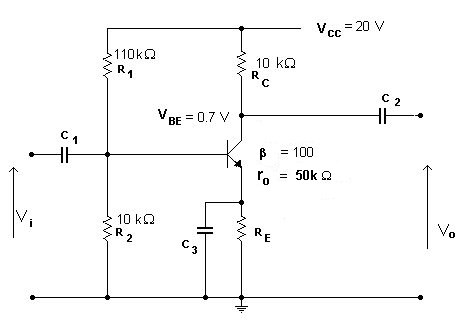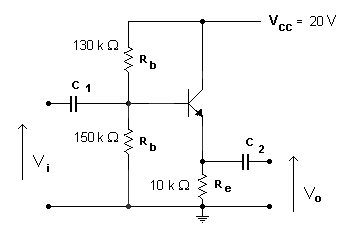EL22C Practice Problems #1   18 February 2016

1. Perform an analysis of the circuit below (draw the equivanent circuit for frequencies where the capacitors can be ignored and calculate the input and output impedances and the voltage gain) and then find a value for the emitter resistor to make the DC collector current 1.2mA2. For the two emitter follower circuits below, calculate the emitter and base currents using a transistor with beta = 200.

Continue your analysis by drawing the equivalent circuit as above and finding the input and output impedances and the voltage gain.EL22C Homepage